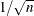# The ARIMA Procedure

### OUTCOV= Data Set

The output data set produced by the OUTCOV= option of the IDENTIFY statement contains the following variables:

• LAG, a numeric variable that contains the lags that correspond to the values of the covariance variables. The values of LAG range from 0 to N for covariance functions and from –N to N for cross-covariance functions, where N is the value of the NLAG= option.

• VAR, a character variable that contains the name of the variable specified by the VAR= option.

• CROSSVAR, a character variable that contains the name of the variable specified in the CROSSCORR= option, which labels the different cross-covariance functions. The CROSSVAR variable is blank for the autocovariance observations. When there is no CROSSCORR= option, this variable is not created.

• N, a numeric variable that contains the number of observations used to calculate the current value of the covariance or cross-covariance function.

• COV, a numeric variable that contains the autocovariance or cross-covariance function values. COV contains the autocovariances of the VAR= variable when the value of the CROSSVAR variable is blank. Otherwise COV contains the cross covariances between the VAR= variable and the variable named by the CROSSVAR variable.

• CORR, a numeric variable that contains the autocorrelation or cross-correlation function values. CORR contains the autocorrelations of the VAR= variable when the value of the CROSSVAR variable is blank. Otherwise CORR contains the cross-correlations between the VAR= variable and the variable named by the CROSSVAR variable.

• STDERR, a numeric variable that contains the standard errors of the autocorrelations. The standard error estimate is based on the hypothesis that the process that generates the time series is a pure moving-average process of order LAG–1. For the cross-correlations, STDERR contains the value, which approximates the standard error under the hypothesis that the two series are uncorrelated.

• INVCORR, a numeric variable that contains the inverse autocorrelation function values of the VAR= variable. For cross-correlation observations (that is, when the value of the CROSSVAR variable is not blank), INVCORR contains missing values.

• PARTCORR, a numeric variable that contains the partial autocorrelation function values of the VAR= variable. For cross-correlation observations (that is, when the value of the CROSSVAR variable is not blank), PARTCORR contains missing values.